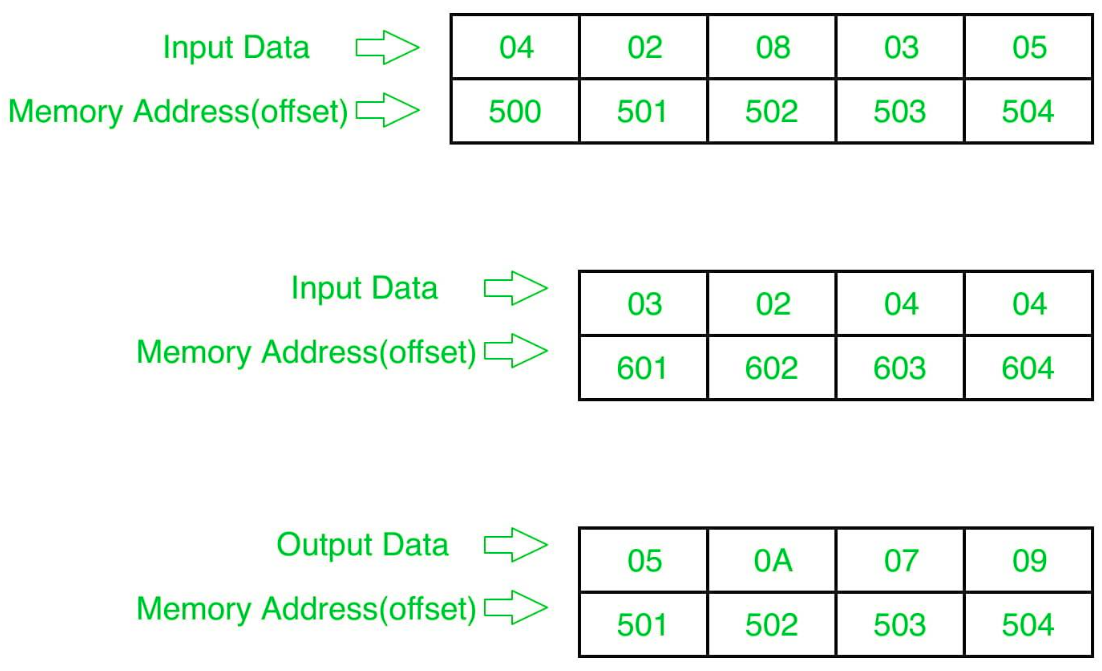Open In App

# 8086 program to determine sum of corresponding elements of two arrays

Problem – Write a program in 8086 microprocessor to find out the sum of two arrays of 8-bit n numbers, where size “n” is stored at offset 500 and the numbers of first array are stored from offset 501 and the numbers of second array are stored from offset 601 and store the result numbers into first array i.e offset 501.

Example –Algorithm –

1. Store 500 to SI and 601 to DI and Load data from offset 500 to register CL and set register CH to 00 (for count).
2. Increase the value of SI by 1.
3. Load first number(value) from next offset (i.e 501) to register AL.
4. Add the value in register AL by value at offset DI.
5. Store the result (value of register AL ) to memory offset SI.
6. Increase the value of SI by 1.
7. Increase the value of DI by 1.
8. Loop above 5 till register CX gets 0.

Program –

400MOV SI, 500SI<-500
403MOV CL, [SI]CL<-[SI]
405MOV CH, 00CH<-00
407INC SISI<-SI+1
408MOV DI, 601DI<-601
40BMOV AL, [SI]AL<-[SI]
40FMOV [SI], ALAL->[SI]
411INC SISI<-SI+1
412INC DIDI<-DI+1
415HLTend

Explanation –

1. MOV SI, 500: set the value of SI to 500
2. MOV CL, [SI]: load data from offset SI to register CL
3. MOV CH, 00: set value of register CH to 00
4. INC SI: increase value of SI by 1.
5. MOV DI, 600: set the value of DI to 600.
6. MOV AL, [SI]: load value from offset SI to register AL
7. ADD AL, [DI]: Add value of register AL by content at offset DI.
8. MOV [SI], AL: store value of register AL at offset SI.
9. INC SI: increase value of SI by 1.
10. INC DI: increase value of DI by 1.